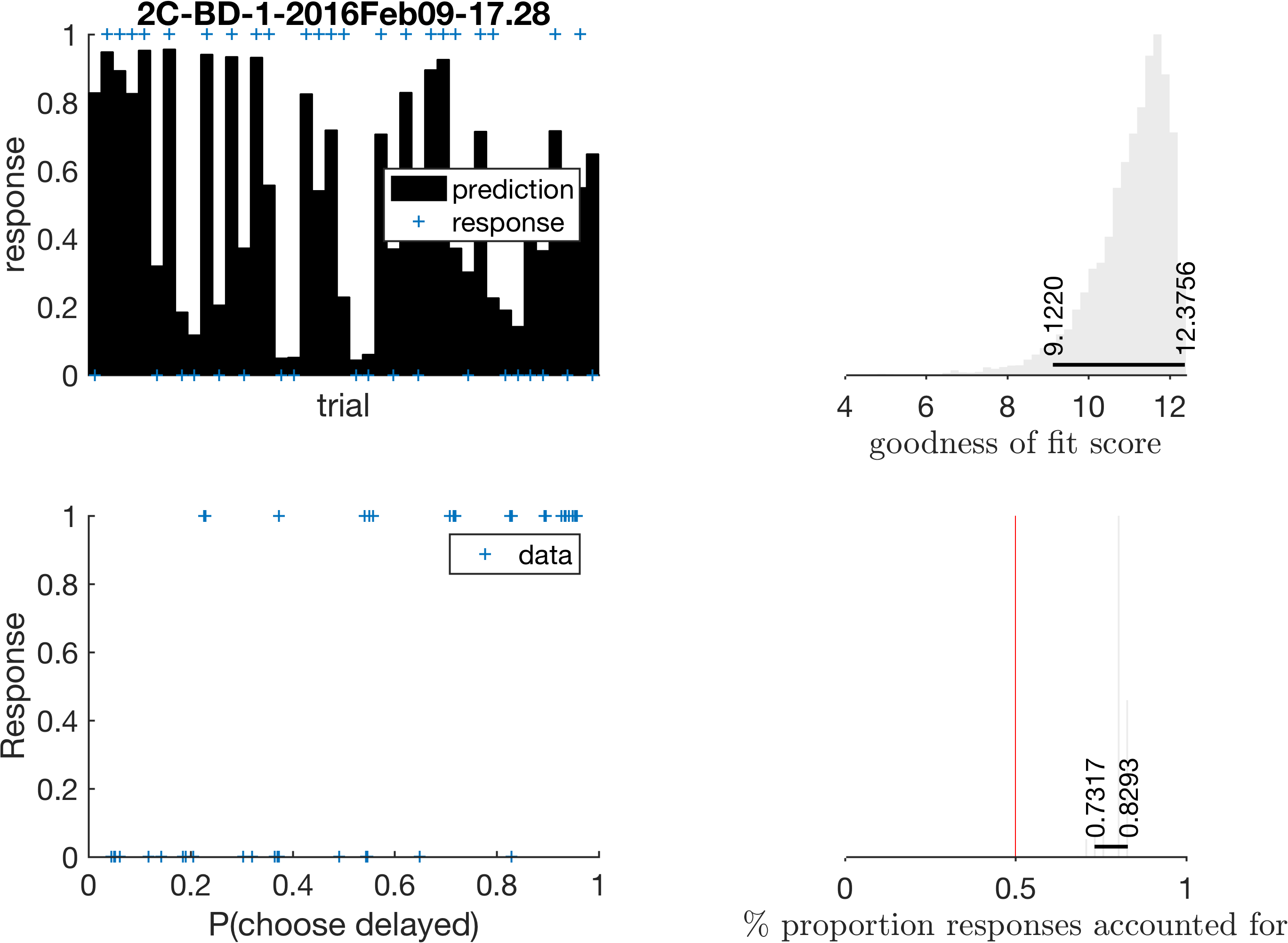# Posterior predictive checks

The toolbox now calculates 2 measures of “goodness of fit” of the models. This is a useful quantitative reassurance that the models describe the participant discounting behaviour better than chance. In turn, this is important when we come to deciding which (if any) data files we should exclude.

Percent responses predicted

The most interpretable measure we calculate is what percentage of each participant’s responses were successfully predicted by the model. We in fact get a distribution over this measure which reflects our confidence in the parameter estimates. In other words, we get a percentage predicted score for every MCMC sample, and therefore uncertainty in parameter estimates will be reflected in uncertainty of model goodness.

I’ve added a new column in the exported parameter estimates called `warning_percent_predicted`. If it is set to 1 (i.e. true) then we should probably exclude this participant. This warning flag is triggered when the 95% highest density intervals overlap with 50% prediction. This amounts to saying that we will exclude participants if the model cannot explain their behaviour better than a control/random response model.Goodness of fit score

I also calculate the log ratio of the probability of the responses under the model verses the control/random response model. Scores around zero imply the model does no better than chance. Currently, this score is calculated, but I use the percent predicted measure more, due to it’s interpretability.

New figure

We also get a new figure, giving some insight into the posterior predictive checks.

• Top left shows responses and predictions as a function of trial.
• Top right shows the distribution of goodness of fit score. Higher scores correspond to better fits.
• Bottom left: possibly not that useful, but response shown as a function of predicted response.
• Bottom right: distribution of proportion responses accounted for. Overlap with 0.5 indicates not better than a control/random preference model.